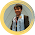### MATH EXERCISE 5

1.   Find the center and radius of the circle ( x2 + y2 + 8x - 8y +23 = 0) on the coordinate  plane.

2.   What is the equation of the circle on the coordinate plane with a radius of 4 and its center at (4, -5)

3.   Evaluate: i4379

4.   Evaluate: (12 + 3i) / (4 – 3i) ; answers: in polar and rectangular forms

5.   Two lines: y = 5x - 10 ; y = 2x - 1 intersect each other on a coordinate plane.
Find their point of intersection.

6.   Convert to Roman Numerals:
a.  3 456
b. 543 342
c. 2 043 598

7.   Convert to Arabic Numerals:
a.  XLVI
b. MDCCXLIX
c. DXXIV

8.   Find the area of the circle inscribed in a square if the area of the latter is 49.

9.   A Sphere with a volume of 36π is inscribed in a Cylinder.
a. Find the Surface Area of the Sphere
b. Find the Surface Area of the Cylinder
c. Find the Volume of the Cylinder
d. Find the height of the Cylinder
e. Find the area of the top of the Cylinder

1.2.1.3.1.